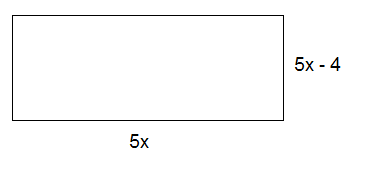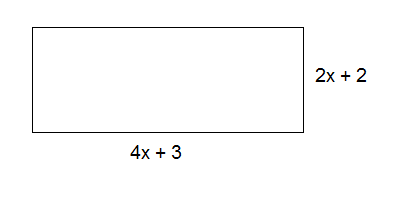# Multiplying polynomials and binomials

We can use the area of a rectangle to explain how you multiply a polynomial by a monomial.

Example

Find the area of this rectangle.$A=b\cdot h$

$A=5x\left ( 5x-4 \right ) \\A=5x\cdot 5x-5x\cdot 4$

$A=5\cdot 5\cdot x\cdot x-5\cdot 4\cdot x$

$A=25x^{2}-20x$

This method is called the distributive property. The distributive property shows us how to write an expression in a different way.

$a(b+c)=ab+ac$

Example

With numbers

$5\left (2+6 \right )=5\cdot 2+5\cdot 6=10+30=40$

With variables and numbers:

$7x+4x= x\left (7+4 \right )=11x$

We can use the area of another rectangle to explain what happens when you multiply two binomials.

ExampleThe area of the rectangle can be calculated by the use of the distributive property:

$A=b\cdot h$

$A=({\color{red} {4x}}+{\color{blue} {3}})({\color{green} {2x}}+2)$

$A=({\color{red} {4x}}+{\color{blue} {3}})\cdot {\color{green} {2x}}+({\color{red} {4x}}+{\color{blue} {3}})\cdot 2$

$A={\color{red} {4x}}\cdot {\color{green} {2x}}+{\color{blue} {3}}\cdot {\color{green} {2x}}+{\color{red} {4x}}\cdot 2+{\color{blue} {3}}\cdot 2$

$A=8x^{2}+6x+8x+6$

$A=8x^{2}+14x+6$

## Video lesson

Expand the expression

$(3x+4)(x^{2}-2)$